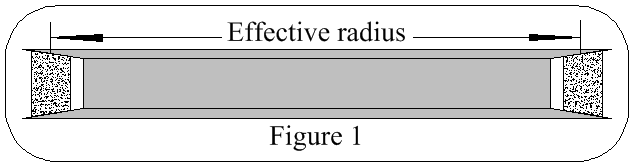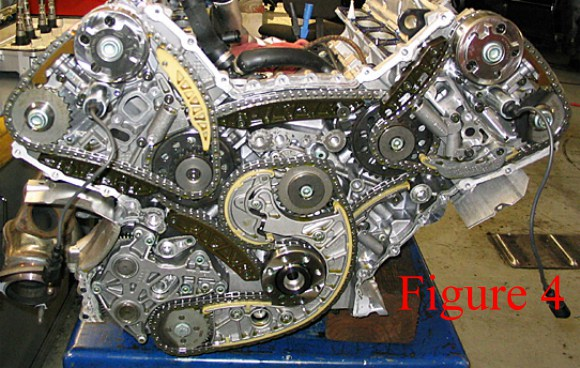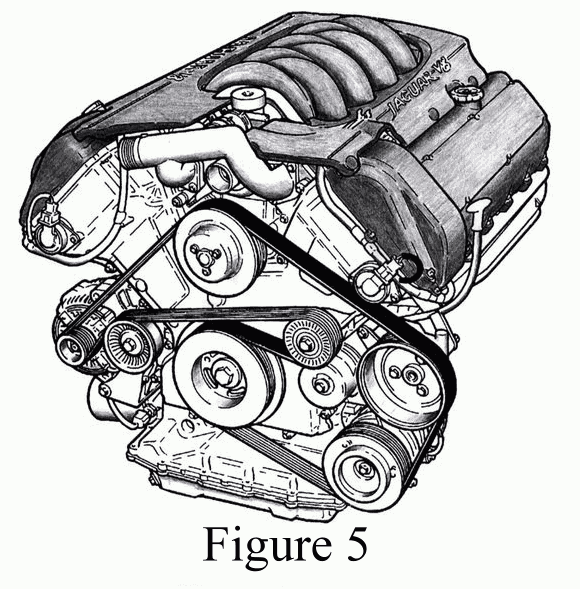# The Length Of Drive Belts

The drive belt is measured at halfway through its radial thickness, as shown in Figure 1.The simplest case is for two pulleys; see Figure 2. In triangle C, x, Rb-Ra: x2 = C2 - (Rb-Ra)2 x = √[C2 - (Rb-Ra)2] a = sin-1(Rb-Ra)/C b = cos-1(Rb-Ra)/ C θa = 360 - 180 - 2a θb = 360 - 2b La = 2.π.Ra.θa/360 Lb = 2.π.Rb.θb/360 Ltotal = 2.x + La + Lb

Most cars thease days have the crankshaft pulley driving an alternator pulley & an air conditioner pulley, as in Figure 3.

α = sin-1(R1-R3)/P1 L1 = cos sin-1(R1-R3)/P1 β = sin-1(R1-R2)/P2 L2 = cos sin-1(R1-R2)/P2 Allowing for O to the longest of the Ls, in this case L2: (The book says O = 0.016P, in this case P2.) 2.E = 2√(O2 + L22/4) = 2√{O2 + [cos sin-1(R1-R2)/P2]2 / 4} γ = sin-1(R3-R2)/P3 L3 = cos sin-1(R3-R2)/P3 ζ = cos-1(P12 + P22 - P32) / 2.P1.P2 Angle between L1 & L2 = ζ + α + β = cos-1(P12 + P22 - P32) / 2.P1.P2 + sin-1(R1-R3)/P1 + sin-1(R1-R2)/P2 R1 contact length = 2.π.R1[cos-1(P12 + P22 - P32) / 2.P1.P2 + sin-1(R1-R3)/P1 + sin-1(R1-R2)/P2] / 360 δ = cos-1(P22 + P32 - P12) / 2.P2.P3 Angle between L2 & L3 = γ + β + δ = sin-1(R3-R2)/P3 + sin-1(R1-R2)/P2 + cos-1(P22 + P32 - P12) / 2.P2.P3 R2 contact length = 2.π.R2[sin-1(R3-R2)/P3 + sin-1(R1-R2)/P2 + cos-1(P22 + P32 - P12) / 2.P2.P3] / 360 ε = cos-1(P12 + P32 - P22) / 2.P1.P3 Angle between L3 & L1 = ε + δ + α = cos-1(P12 + P32 - P22) / 2.P1.P3 + cos-1(P22 + P32 - P12) / 2.P2.P3 + sin-1(R1-R3)/P1 R3 contact length = 2.π.R3[cos-1(P12 + P32 - P22) / 2.P1.P3 + cos-1(P22 + P32 - P12) / 2.P2.P3 + sin-1(R1-R3)/P1] / 360 So the belt length = cos sin-1(R1-R3)/P1 + 2√{O2 + [cos sin-1(R1-R2)/P2]2 / 4} + cos sin-1(R3-R2)/P3 + 2.π.R1[cos-1(P12 + P22 - P32) / 2.P1.P2 + sin-1(R1-R3)/P1 + sin-1(R1-R2)/P2] / 360 + 2.π.R2[sin-1(R3-R2)/P3 + sin-1(R1-R2)/P2 + cos-1(P22 + P32 - P12) / 2.P2.P3] / 360 + 2.π.R3[cos-1(P12 + P32 - P22) / 2.P1.P3 + cos-1(P22 + P32 - P12) / 2.P2.P3 + sin-1(R1-R3)/P1] / 360

Simple. Now you've got the hang of it, try the belt & chains in figures 4 & 5.Some notes on belt drives Tq Torque on the driven shaft Tq = (T1 - T2).Dn / 2 Power = (T1 - T2).N.DN / 2 Belt slip is a function of total tension & differential tension. Efficiency = A + BeC.Tq + D.Tq A, B, C & D are constants, +ve or -ve real numbers. Traction coefficient is an indicator of how hard a belt is working.

Howard's Stuff Index

Back to Pigeon's Nest

Be kind to pigeons